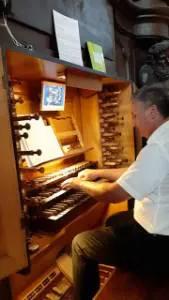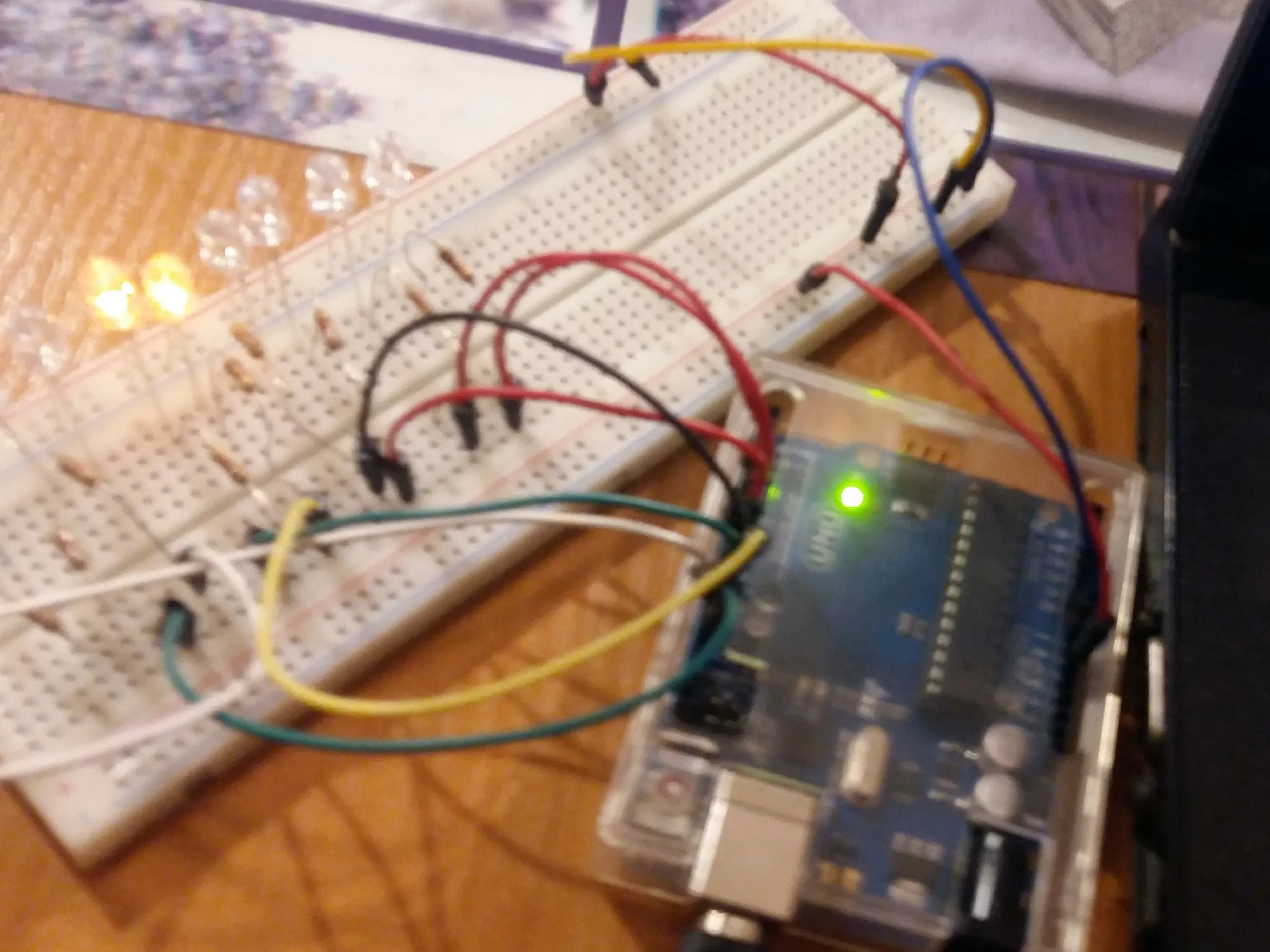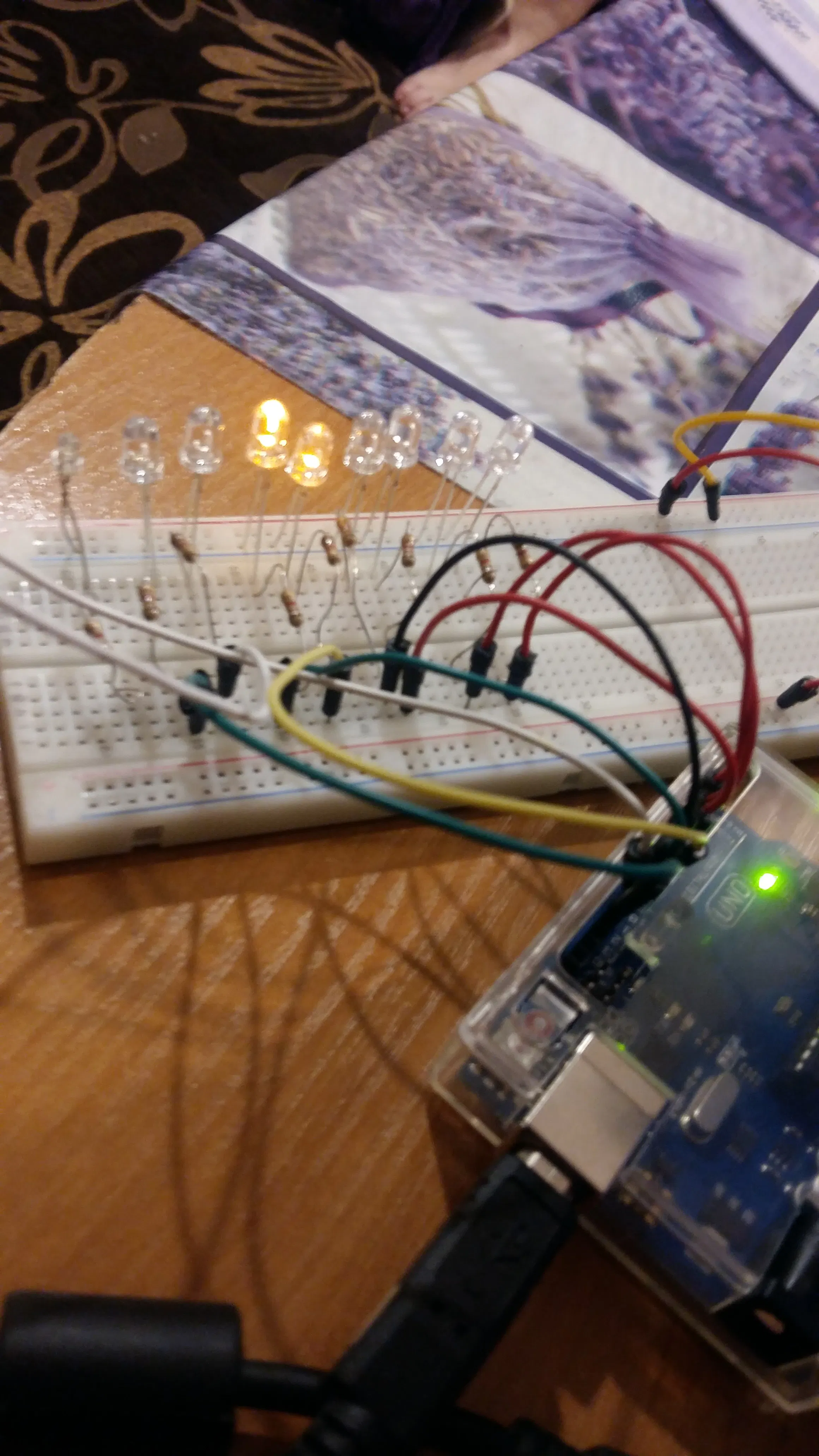# Small MIDI decoder on the Arduino UNO for Hauptwerk

The small MIDI decoder was constructed to support LED indicators for stops switches in software GrandOrgue or Hauptwerk (VPO).

BeginnerProtip253## Things used in this project

### Hardware componentsArduino UNO & Genuino UNO
×1
 Board
×1LED (generic)
×18Resistor 1k ohm
×18

### Software apps and online servicesArduino IDE
 AvrDudess

### Hand tools and fabrication machines

 programmer USB Asp

## Schematics

### Viev## Code

### Kod for Small Dekoder MIDI - Arduino UNO for GrandOrgue or Hauptwerk

C/C++
```// Small dekoder Midi - R. Milewski
#include <MIDI.h>   // Add Midi Library

//#define LED 13
//#define LED2 12// Arduino Board LED is on Pin 13

//Create an instance of the library with default name, serial port and settings
MIDI_CREATE_DEFAULT_INSTANCE();

void setup() {

pinMode (A0, OUTPUT); // Set Arduino board analog pins to output
digitalWrite (A0, LOW);
pinMode (A1, OUTPUT);
digitalWrite (A1, LOW);
pinMode (A2, OUTPUT);
digitalWrite (A2, LOW);
pinMode (A3, OUTPUT);
digitalWrite (A3, LOW);
pinMode (A4, OUTPUT);
digitalWrite (A4, LOW);
pinMode (A5, OUTPUT);

for (int a=2; a<14; a++)
{
pinMode (a, OUTPUT); // Set Arduino board digital pins to output
digitalWrite (a, LOW); // Set Arduino board digitsl pins to KIGH

}

// self-test
for (int a=2; a<14; a++)
{
digitalWrite (a, HIGH); // Set Arduino board digital pins to HIGH
delay(250);
digitalWrite (a, LOW); // Set Arduino board digital pins to LOW
delay(50);
}

MIDI.begin(MIDI_CHANNEL_OMNI); // Initialize the Midi Library.
// OMNI sets it to listen to all channels.. MIDI.begin(2) would set it
// to respond to notes on channel 2 only.
MIDI.turnThruOff(); // Turn off loop Midi IN to OUT
MIDI.setHandleNoteOn(MyHandleNoteOn); // This is important!! This command
// tells the Midi Library which function you want to call when a NOTE ON command
// is received. In this case it's "MyHandleNoteOn".
MIDI.setHandleNoteOff(MyHandleNoteOff); // This command tells the Midi Library
// to call "MyHandleNoteOff" when a NOTE OFF command is received.
}

void loop() { // Main loop
}

// MyHandleNoteON is the function that will be called by the Midi Library
// when a MIDI NOTE ON message is received.
// It will be passed bytes for Channel, Pitch, and Velocity
void MyHandleNoteOn(byte channel, byte pitch, byte velocity) {
switch (pitch) {
case 54+34:
digitalWrite (A0, HIGH);
break;
case 55+34:
digitalWrite (A1, HIGH);
break;
case 56+34:
digitalWrite (A2,HIGH);
break;
case 57+34:
digitalWrite (A3, HIGH);
break;
case 58+34:
digitalWrite (A4, HIGH);
break;
case 59+34:
digitalWrite (A5, HIGH);
break;

default:
// if nothing else matches, do the default
// default is optional
break;
}
for (int a=2; a<14; a++)
{
if (pitch == 58+a) {
digitalWrite (a, HIGH); // Set Arduino board pin 13 to output
}
}

}

// MyHandleNoteOFF is the function that will be called by the Midi Library
// when a MIDI NOTE OFF message is received.
// * A NOTE ON message with Velocity = 0 will be treated as a NOTE OFF message *
// It will be passed bytes for Channel, Pitch, and Velocity
void MyHandleNoteOff(byte channel, byte pitch, byte velocity) {
switch (pitch) {
case 54+34:
digitalWrite (A0, LOW);
break;
case 55+34:
digitalWrite (A1, LOW);
break;
case 56+34:
digitalWrite (A2, LOW);
break;
case 57+34:
digitalWrite (A3, LOW);
break;
case 58+34:
digitalWrite (A4, LOW);
break;
case 59+34:
digitalWrite (A5, LOW);
break;

default:
// if nothing else matches, do the default
// default is optional
break;
}

for (int a=2; a<14; a++)
{
if (pitch == 58+a) {
digitalWrite (a, LOW); // Set Arduino board pin 13 to output
}
}

}
```

### Kod for Decoder MIDI Arduino Mega for GrandOrgue or Hauptwerk

C/C++
```// Kod for Decoder MIDI  Arduino Mega for GrandOrgue or Hauptwerk
#include <MIDI.h>   // Add Midi Library

//#define LED 13
//#define LED2 12// Arduino Board LED is on Pin 13

//Create an instance of the library with default name, serial port and settings
MIDI_CREATE_DEFAULT_INSTANCE();

void setup() {

pinMode (A0, OUTPUT); // Set Arduino board analog pins to output
digitalWrite (A0, HIGH);
pinMode (A1, OUTPUT);
digitalWrite (A1, HIGH);
pinMode (A2, OUTPUT);
digitalWrite (A2, HIGH);
pinMode (A3, OUTPUT);
digitalWrite (A3, HIGH);
pinMode (A4, OUTPUT);
digitalWrite (A4, HIGH);
pinMode (A5, OUTPUT);
digitalWrite (A5, HIGH);
pinMode (A6, OUTPUT);
digitalWrite (A6, HIGH);
pinMode (A7, OUTPUT);
digitalWrite (A7, HIGH);
pinMode (A8, OUTPUT);
digitalWrite (A8, HIGH);
pinMode (A9, OUTPUT);
digitalWrite (A9, HIGH);
pinMode (A10, OUTPUT);
digitalWrite (A10, HIGH);
pinMode (A11, OUTPUT);
digitalWrite (A11, HIGH);
pinMode (A12, OUTPUT);
digitalWrite (A12, HIGH);
pinMode (A13, OUTPUT);
digitalWrite (A13, HIGH);
pinMode (A14, OUTPUT);
digitalWrite (A14, HIGH);
pinMode (A15, OUTPUT);
digitalWrite (A15, HIGH);

for (int a=2; a<54; a++)
{
pinMode (a, OUTPUT); // Set Arduino board digital pins to output
digitalWrite (a, HIGH); // Set Arduino board digitsl pins to KIGH

}

// self-test
for (int a=14; a<36; a++)
{
digitalWrite (a, LOW); // Set Arduino board digital pins to HIGH
delay(250);
digitalWrite (a, HIGH); // Set Arduino board digital pins to LOW
delay(50);
}

MIDI.begin(MIDI_CHANNEL_OMNI); // Initialize the Midi Library.
// OMNI sets it to listen to all channels.. MIDI.begin(2) would set it
// to respond to notes on channel 2 only.
MIDI.setHandleNoteOn(MyHandleNoteOn); // This is important!! This command
// tells the Midi Library which function you want to call when a NOTE ON command
// is received. In this case it's "MyHandleNoteOn".
MIDI.setHandleNoteOff(MyHandleNoteOff); // This command tells the Midi Library
// to call "MyHandleNoteOff" when a NOTE OFF command is received.
}

void loop() { // Main loop
}

// MyHandleNoteON is the function that will be called by the Midi Library
// when a MIDI NOTE ON message is received.
// It will be passed bytes for Channel, Pitch, and Velocity
void MyHandleNoteOn(byte channel, byte pitch, byte velocity) {
switch (pitch) {
case 54+34:
digitalWrite (A0, LOW);
break;
case 55+34:
digitalWrite (A1, LOW);
break;
case 56+34:
digitalWrite (A2, LOW);
break;
case 57+34:
digitalWrite (A3, LOW);
break;
case 58+34:
digitalWrite (A4, LOW);
break;
case 59+34:
digitalWrite (A5, LOW);
break;
case 60+34:
digitalWrite (A6, LOW);
break;
case 61+34:
digitalWrite (A7, LOW);
break;
case 62+34:
digitalWrite (A8, LOW);
break;
case 63+34:
digitalWrite (A9, LOW);
break;
case 64+34:
digitalWrite (A10, LOW);
break;
case 65+34:
digitalWrite (A11, LOW);
break;
case 66+34:
digitalWrite (A12, LOW);
break;
case 67+34:
digitalWrite (A13, LOW);
break;
case 68+34:
digitalWrite (A14, LOW);
break;
case 69+34:
digitalWrite (A15, LOW);
break;

default:
// if nothing else matches, do the default
// default is optional
break;
}
for (int a=2; a<54; a++)
{
if (pitch == 34+a) {
digitalWrite (a, LOW); // Set Arduino board pin 13 to output
}
}

}

// MyHandleNoteOFF is the function that will be called by the Midi Library
// when a MIDI NOTE OFF message is received.
// * A NOTE ON message with Velocity = 0 will be treated as a NOTE OFF message *
// It will be passed bytes for Channel, Pitch, and Velocity
void MyHandleNoteOff(byte channel, byte pitch, byte velocity) {
switch (pitch) {
case 54+34:
digitalWrite (A0, HIGH);
break;
case 55+34:
digitalWrite (A1, HIGH);
break;
case 56+34:
digitalWrite (A2, HIGH);
break;
case 57+34:
digitalWrite (A3, HIGH);
break;
case 58+34:
digitalWrite (A4, HIGH);
break;
case 59+34:
digitalWrite (A5, HIGH);
break;
case 60+34:
digitalWrite (A6, HIGH);
break;
case 61+34:
digitalWrite (A7, HIGH);
break;
case 62+34:
digitalWrite (A8, HIGH);
break;
case 63+34:
digitalWrite (A9, HIGH);
break;
case 64+34:
digitalWrite (A10, HIGH);
break;
case 65+34:
digitalWrite (A11, HIGH);
break;
case 66+34:
digitalWrite (A12, HIGH);
break;
case 67+34:
digitalWrite (A13, HIGH);
break;
case 68+34:
digitalWrite (A14, HIGH);
break;
case 69+34:
digitalWrite (A15, HIGH);
break;

default:
// if nothing else matches, do the default
// default is optional
break;
}

for (int a=2; a<54; a++)
{
if (pitch == 34+a) {
digitalWrite (a, HIGH); // Set Arduino board pin 13 to output
}
}

}
```

## Credits

### Romekmil

5 projects • 2 followers